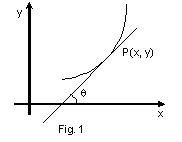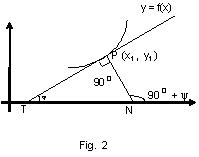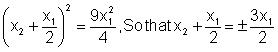Click to Chat

1800-1023-196

+91-120-4616500

CART 0

• 0

MY CART (5)

Use Coupon: CART20 and get 20% off on all online Study Material

ITEM
DETAILS
MRP
DISCOUNT
FINAL PRICE
Total Price: Rs.

There are no items in this cart.
Continue Shopping```Geometrical Meaning of Derivative at Point

The derivative [f'(x) or dy/dx] of the function y = f(x) at the point P(x, y) (when exists) is equal to the slope (or gradient) of the tangent line to the curve y = f(x) at P(x, y).

Slope of tangent to the curve y = f(x) at the point (x, y) is m = tanθ = [dy/dx](x,y)Equation of Tangent

The equation of tangent to the curve

y = f(x) at the point P(x1, y1) is given by y – y1 = [dy/dx](x,y) (x – x1)Notes:

(i) If dy/dx = 0 then the tangent to curve y = f(x) at the point (x, y) is parallel to the x-axis.

(ii) If dy/dx –> ∞, dx/dy = 0, then the tangent to the curve y = f(x) at the point (x, y) is parallel to the y-axis.

(iii) If dy/dx = tanθ > 0, then the tangent to the curve y = f(x) at the point (x, y) makes an acute angle with positive x-axis and vice versa.

Equation of Normal

The normal to the curve at the point P(x1, y1) is a line perpendicular to the tangent at the point P(x1, y1) and passing through it. The angle between a tangent and a normal at a point is always 900. The equation of the normal to the curve y=f(x) at a given point P(x1, y1)is given by(x - x1) + (y - y1) [dy/dx](x1,y1)  = 0.

Equation of Tangent and Normal If Equation of the Curve is given in Parametric form

If the equation of the curve is in the parametric form x = f(t) and y = g(t), then the equations of the tangent and the normal are

y – g(t) = g'(t)/f'(t)(x–f(t)) and f'(t)[x–f(t)] + g'(t) [y–g(t)] = 0 and  respectively

Illustration 9: If at each point of the curve y = x3 – ax2 + x + 1 the tangents is inclined at an acute angle with the positive direction of the x-axis, then find the interval in which a lies.

Key concept: since the tangent is always inclined at an acute angle with the x-axis, hence and the use the concept of quadratic equation that ax2 + bx + c > 0 for all x ÎR if a > 0 and D < 0

Solution:             y = x3 – ax2 + x + 1 and the tangent is inclined at an acute angle with the positive direction of x-axis,

=> dx/dy > 0             ∴ 3x2 – 2ax + 1 >0, for all x ∈ R

=> (2a)2 – 4 (3)(1) < 0 => 4(a2  – 3)< 0 => (a – √3) (a + √3) < 0

∴ – √3 < a < √3

Illustration 10:   The tangent to the curve y = x – x3 at a point P meets the curve again at Q. Prove that one point of trisection of PQ lies on y-axis. Find the locus of the other point of trisection.

Solution:             For y = x – x3, dy/dx = 1 – 3x2

Hence the equation of the tangent at the point P(x1, y1) is;

y – y1= (1 – 3x12)(x – x1)

It meets the curve again at Q (x2, y2)

Hence, (x2– x23)– (x1 – x13) = (1 – 3x12)(x2 – x1)

=> (x2 – x1)[1- (x22 + x1x2 + x12)] = (x2 – x1)(1 – 3x12)

=> 1 – x22– x1x2– x12 = 0

orSince x1 ¹ x2, we have x2 = - 2x1

Q is (-2x1, - 2x1 + 8x13)

If L1(α, β) is the point of trisection of PQ then α = 2x1 – 2x1 / 3 = 0. Hence L1lies on the y-axis. If L2(h, k) is the other point of trisection, then h = x1 4x1 /3 = –x1 and k = y1–4x1 + 16x13/3

i.e. k = y1 –4x1 + 16x13/ 3 = –x1 + 5x13

k = h - 5h3

∴ locus of (h, k) is y = x – 5x3.

Angle of Intersections of two Curves

Let C1: y= f(x) and C2: y = g(x) be two curves. If two curves intersect at point P (x1, y1). Then the angle of intersection of two curves is defined as the angles between the tangents at their intersection. And is given by tan θ = f'(x1) – g'(x) / 1 + f'(x1)g'(x1)

Note:

(i) If the curves touch at P, then θ = 0 so that f ’ (x1) = g ‘ (x1)

(ii) If the angle θ = 90o (πc/2, right angle) i.e. Two tangents are perpendicular to each other then the curves are said to cut orthogonally, then f ‘(x1). g ’(x1) =- 1.

Illustration 11:   If the curves x2-4y2+c=0 and y2 = 4x intersect orthogonally then find the range of c.

Solution: Curves will intersect if x2 – 16 x + c = 0 has real roots. Thus c £ 64.

For x2 – 4y2 + c = 0, dy/dx = x/4y

For y2 = 4x, dy/dx = 2/y

If curves intersect orthogonally then x/4y.2/y = –1 => x = –2y2 = –8x

=> x = 0 => y = 0. But if y = 0, slope of both curves undefined.
```### Course Features

• 731 Video Lectures
• Revision Notes
• Previous Year Papers
• Mind Map
• Study Planner
• NCERT Solutions
• Discussion Forum
• Test paper with Video Solution